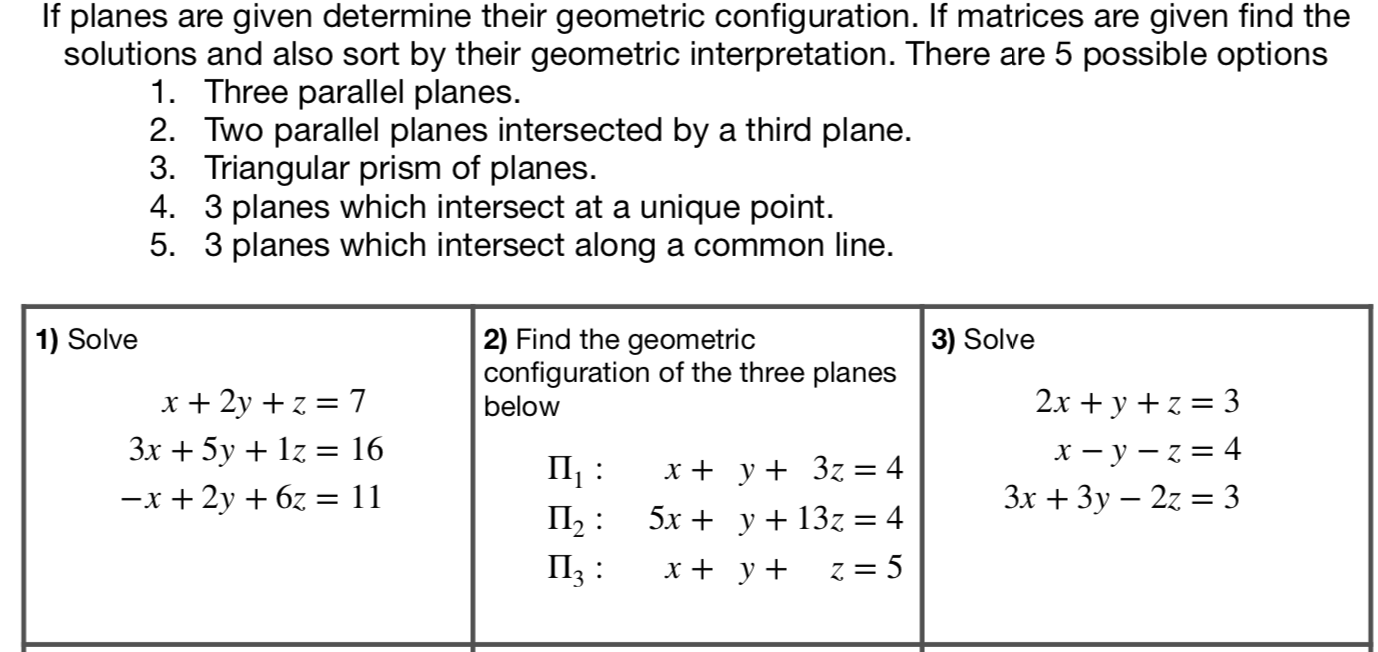Categories

# Geometric Interpretation of Simultaneous Equations

One of the topics requested by my current Year 13 Further Mathematicians in their revision lessons has been the geometric interpretation of the solution of simultaneous equations.

I hadn’t taught this at A-Level until the new specification so it was interesting to teach. I would always have done this using augmented matrices, performing row operations and then looking at the difference in rank between the matrix and the augmented matrix so the approach at A-Level was new to me.The idea of the activity is to sort the 12 given situations into the five geometric possibilities.

File for use available here.

## 2 replies on “Geometric Interpretation of Simultaneous Equations”Damian Rogansays:

In Q11 the equation of the 3rd plane is half obscured as it doesn’t fit in the box!tombennisonsays:

Hi,
This should be fixed now too.
Tom